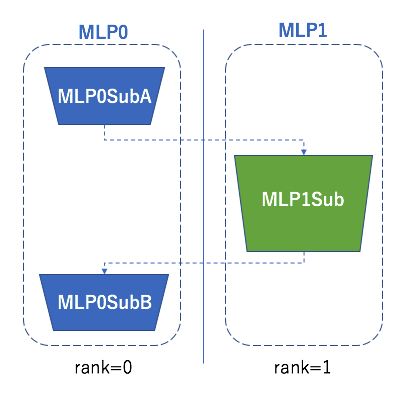# Example 1: Simple MLP¶

Here is the first example of model parallel, a simple MLP separated on two processes.First, let’s create a ChainerMN communicator:

if args.gpu:
comm = chainermn.create_communicator('pure_nccl')
device = comm.intra_rank
else:
comm = chainermn.create_communicator('naive')
device = -1


As we saw in Model Parallel on ChainerMN, one naive implementation would be to use the point-to-point communication such as send and recv:

class MLP0(chainer.Chain):
def __init__(self, comm, n_out):
super(MLP0SubA, self).__init__(
l1=L.Linear(784, n_out))

def __call__(self, x):
h0 = F.relu(self.l1(x))
phi = chainermn.functions.send(h0, self.comm, rank=1)
# Note: do not forget to pass delegate variable
y = chainermn.functions.recv(self.comm, rank=1, delegate_variable=phi)
return y

class MLP1(chainer.Chain):
def __init__(self, n_units, n_out):
super(MLP1Sub, self).__init__(
l2=L.Linear(None, n_units),
l3=L.Linear(None, n_out))

def __call__(self, _):
h0 = chainermn.functions.recv(self.comm, rank=0)
h1 = F.relu(self.l2(h0))
return chainermn.functions.send(self.l3(h1), self.comm, rank=0)


One should note that

• MLP0: delegate variable is indispensable which is passed from send to recv.

• MLP1: the return value from send must be returned in __call__, which is used to track back the computational graph.

On each process, different models are trained:

if comm.rank == 0:
model = L.Classifier(MLP0(comm, 100))
elif comm.rank == 1:
model = MLP1(comm, 100, 10)


Since MLP1 receives its inputs from MLP0 over the point-to-point communication, let’s use empty_dataset instead of the usual dataset:

# Iterate dataset only on worker 0.
train, test = chainer.datasets.get_mnist()
if comm.rank == 1:
train = chainermn.datasets.create_empty_dataset(train)
test = chainermn.datasets.create_empty_dataset(test)


Now we can run a model parallel architecture.

There is an alternative API to define the same model without explicitly defining communication paths:

class MLP0SubA(chainer.Chain):
def __init__(self, comm, n_out):
super(MLP0SubA, self).__init__(
l1=L.Linear(784, n_out))

def __call__(self, x):
return F.relu(self.l1(x))

class MLP0SubB(chainer.Chain):
def __init__(self, comm):
super(MLP0SubB, self).__init__()

def __call__(self, y):
return y

class MLP0(chainermn.MultiNodeChainList):
# Model on worker 0.
def __init__(self, comm, n_out):
super(MLP0, self).__init__(comm=comm)

class MLP1Sub(chainer.Chain):
def __init__(self, n_units, n_out):
super(MLP1Sub, self).__init__(
l2=L.Linear(None, n_units),
l3=L.Linear(None, n_out))

def __call__(self, h0):
h1 = F.relu(self.l2(h0))
return self.l3(h1)

class MLP1(chainermn.MultiNodeChainList):
# Model on worker 1.
def __init__(self, comm, n_units, n_out):
super(MLP1, self).__init__(comm=comm)

MultiNodeChainList enables to define a multi model architecture, by adding non-connected component with add_link. Two arguments rank_in and rank_out specifies from which process the added link receives their inputs, and to which process it sends their outputs.